## Cantor never bores (1)

Given a set of countable sets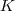, such thatis totally ordered by inclusion, videlicet for every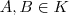either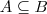or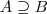. Intuitively, for at every step in this chain one element at least must be added, one expects the setto be countable as well.

Supposeis countable. Then the union,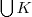is a countable union of countable sets, hence countable. (Suppose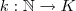is an enumeration ofandenumerations of the elements of the chain. Thenenumerates.)

Thusis an upper bound of. In the poset of countable subsets of some set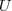, of whichis a subset, every non-empty chain has an upper bound. Hence, using Zorn’s lemma there is a maximal element, say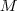.

Supposeis uncountable, then there exists a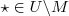.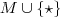is most definitely also countable and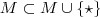which contradicts’s maximality. We are forced to conclude that there exists an uncountable chain of countable sets.

Cantor’s set theory keeps surprising.

Update: an example of such a chain is the set of the countable ordinals.

Another update: a “more concrete” example are the downsets in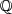without the empty set anditself. These downsets correspond to real numbers, see Dedekind Cuts.

## Aperitif for order

Assign 1 to True and 0 to False. Now the minimum corresponds to “and” and maximum to “or”. If you give it a bit more though, less or equal to corresponds to implication. This is a lot more general than this specific case. Add .5 for a third value (eg. NULL) and it still yields natural results.

We can recognize the behaviour of minima and maxima in a lot of other things. Take for instance set inclusion as order with intersection as minimum and union as maximum. Actually, the link between general order and set inclusion is frequently made to then propose that “intersection of two set of cases” and “logical and” do look a lot alike.

This is just the tip of the huge iceberg. Order appears everywhere! Everywhere in Math. Everywhere in CS. And its just recognizing simple order I’ve demonstrated. Other useful concepts in order theory that I didn’t even touch are Galois Connections and formal concept analysis.

Oh, another example of an order are integers with bitwise or and bitwise and. It is left as an exercise to the reader when one integer is greater than another.

Interested? Buy an Introduction to Lattices and Order.